# Exercise 6.1 class 9 NCERT solutions for maths chapter 6

In this page we have NCERT solutions for class 9 maths chapter 6 Lines and Angles for exercise 6.1.

## Exercise 6.1 class 9 math chapter 6 solutions

Question 1
In below figure, lines AB and CD intersect at O. If ∠AOC + ∠BOE = 70° and ∠BOD = 40°, find ∠BOE and reflex ∠COE.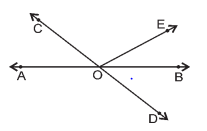Given,
∠AOC + ∠BOE = 70° and ∠BOD = 40°
Now AOB is a straight line
∠AOC + ∠BOE +∠COE = 180°
70° +∠COE = 180°
∠COE = 110°
Also COD is a straight lne
∠COE +∠BOD + ∠BOE = 180°
110° +40° + ∠BOE = 180°
150° + ∠BOE = 180°
∠BOE = 30°

Question 2
In below figure lines XY and MN intersect at O. If ∠POY = 90° and a : b = 2 : 3, find c.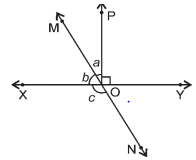Given,
∠POY = 90° and a : b = 2 : 3
Lt x be the common ration, then a=2x and b=3x
Now XOY is a straight line
∠POY + a + b = 180°
90° + a + b = 180°
a + b = 90°
2x + 3x = 90°
5x = 90°
x = 18°
So a = 2×18° = 36°
and b = 3×18° = 54°
Also, now angle b and Angle c forms a linear pair
b + c = 180°
54° + c = 180°
c = 126°

Question 3
In below figure, ∠PQR = ∠PRQ, then prove that ∠PQS = ∠PRT.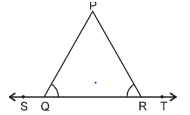Given
∠PQR = ∠PRQ
To prove,
∠PQS = ∠PRT
Now   ∠PQR and ∠PQS forms a linear pair
∠PQR +∠PQS = 180°
∠PQS = 180° - ∠PQR --- (a)
Also, ∠PRQ and ∠PRT forms a linear pair
∠PRQ +∠PRT = 180° ( ∠PRT = 180° - ∠PRQ
Now as (∠PQR = ∠PRQ
∠PRQ = 180° - ∠PQR --- (b)
From (a) and (b)
∠PQS = ∠PRT = 180° - ∠PQR
Therefore, ∠PQS = ∠PRT

Question 4
In below figure, if x + y = w + z, then prove that AOB is a line.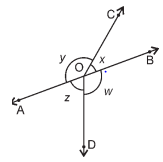Given,
x + y = w + z
To Prove,
AOB is a line or x + y = 180°
Now O is the point and we have four angles around it
x + y + w + z = 360°
(x + y) + (w + z) = 360° Now Given x + y = w + z
(x + y) + (x + y) = 360°
2(x + y) = 360°
(x + y) = 180°
Hence, x + y makes a linear pair. Therefore, AOB is a straight line.

Question 5
In below figure, POQ is a line. Ray OR is perpendicular to line PQ. OS is another ray lying between rays OP and OR. Prove that ∠ROS = 1/2(∠QOS – ∠POS).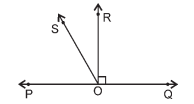Given,
OR is perpendicular to line PQ
To prove,
∠ROS = 1/2(∠QOS – ∠POS)
Proof
∠POR = ∠ROQ = 90° (Perpendicular)
From the figure, it is clear that
∠QOS = ∠ROQ + ∠ROS = 90° + ∠ROQ --- (a)
∠POS = ∠POR - ∠ROS = 90° - ∠ROQ --- (b)
Subtracting (b) from (a)
∠QOS - ∠POS = 90° + ∠ROQ - (90° - ∠ROQ)
⇒ ∠QOS - ∠POS = 90° + ∠ROQ - 90° + ∠ROQ
⇒ ∠QOS - ∠POS = 2∠ROQ
⇒ ∠ROS = 1/2(∠QOS – ∠POS)
Hence, proved.

Question 6
It is given that ∠XYZ = 64° and XY is produced to point P. Draw a figure from the given information. If ray YQ bisects ∠ZYP, find ∠XYQ and reflex ∠QYP.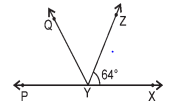Given,
∠XYZ = 64°
YQ bisects ∠ZYP Now angle ∠XYZ and ∠ZYP forms a linear pair
∠XYZ +∠ZYP = 180°
64° +∠ZYP = 180° ∠ZYP = 116°
Now YQ bisects ∠ZYP
∠ZYQ = ∠QYP
Also ∠ZYP = ∠ZYQ + ∠QYP So ∠ZYP = 2∠ZYQ 2∠ZYQ = 116°
∠ZYQ = 58° = ∠QYP
Now,
∠XYQ = ∠XYZ + ∠ZYQ ∠XYQ = 64° + 58°
∠XYQ = 122°
Also,
reflex ∠QYP = 180° + ∠XYQ
∠QYP = 180° + 122°
=3020

### class 9 math 6.1 Summary

1. NCERT solutions for class 9 maths chapter 6 Lines and Angle Exercise 6.1 has been prepared by Expert with utmost care. If you find any mistake.Please do provide feedback on mail. You can download the solutions as PDF in the below Link also
2. This chapter 6 has total 3 Exercise 6.1 ,6.2 and 6.3. This is the First exercise in the chapter.